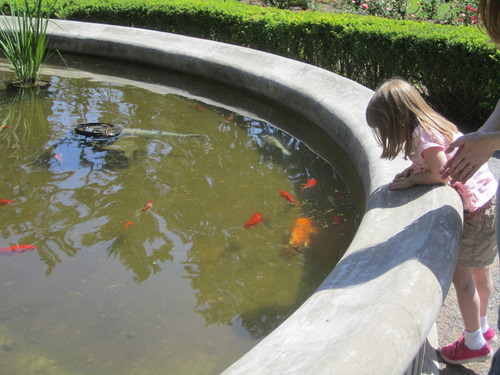# 6.8: Radius or Diameter of a Circle Given Area

•• Contributed by CK12
• CK12

To find the radius, divide the area by pi, then take the square root.Figure $$\PageIndex{1}$$

Clara took her little sister, Grace, to the fish pond at the local park. Grace saw a penny in the center of the pond and wanted Clara to reach it for her. A sign stated that the area of the pond was 113.04 sq. ft. Can Clara reach the penny without falling in?

In this concept, you will learn how to find the radius (and diameter) of a circle if you know its area.

## Finding the Radius or Diameter of a Circle Given Area

The formula for the area of a circle, $$A=\pi r^2$$, can also be used to solve for the radius and diameter.

Let’s look an example.

The area of a circle is 113.04 square inches. What is its radius?

First, write the formula.

$$A=\pi r^2$$

Next, substitute in what you know.

$$113.04=(3.14)r^2$$

Next, begin isolating the r by dividing both sides of the equation by 3.14.

$$36=r^2$$

Then, take the square root of both sides.

$$6=r$$

The answer is r=6. The radius of the circle is 6 inches.

Example $$\PageIndex{1}$$

Earlier, you were given a problem about Clara and Grace, who were at the circular 113.04 sq. ft. fish pond.

Clara wondered if she could reach a penny in the middle without falling in.

Solution

First, write the formula.

$$A=\pi r^2$$

Next, substitute in what you know.

$$113.04=(3.14)r^2$$

Then, begin isolating the r by dividing both sides of the equation by 3.14.

$$36=r^2$$

Take the square root of both sides.

$$6=r$$

The answer is r=6. The radius of the circle is 6 feet. Unless Clara wants to swim with the goldfish, she’d best leave the penny where it is!

Example $$\PageIndex{2}$$

What is the diameter of a circle if its area is $$379.94 cm^2$$?

Solution

First, write the formula.

$$A=\pi r^2$$

Next, substitute in what you know.

$$379.94=(3.14)r^2$$

Next, begin isolating the r by dividing both sides of the equation by 3.14.

$$121=r^2$$

Then, take the square root of both sides.

$$11=r$$

Remember that you are solving for the diameter.

\begin{aligned} d&=2r \\ d&=2\times 11 \\ d&=22\end{aligned}

The answer is the diameter, d=22 cm.

Example $$\PageIndex{3}$$

Solve for the radius of a circle if area=153.86 sq. in.

Solution

First, write the formula.

$$A=\pi r^2$$

Next, substitute in what you know.

$$153.86=(3.14)r^2$$

Next, begin isolating the r by dividing both sides of the equation by 3.14.

$$49=r^2$$

Then, take the square root of both sides.

$$7=r$$

The answer is $$r=7$$. The radius of the circle is 7 inches.

Example $$\PageIndex{4}$$

Find the radius of a circle with an area of 379.94 sq. ft.

Solution

First, write the formula.

$$A=\pi r^2$$

Next, substitute in what you know.

$$379.94=(3.14)r^2$$

Then, begin isolating the r by dividing both sides of the equation by 3.14.

$$121=r^2$$

Take the square root of both sides.

$$11=r$$

The answer is r=11. The radius of the circle is 11 feet.

Example $$\PageIndex{5}$$

The area of a circle is 452.16 sq. m. Find its radius.

Solution

First, write the formula.

$$A=\pi r^2$$

Next, substitute in what you know.

$$452.16=(3.14)r^2$$

Then, begin isolating the r by dividing both sides of the equation by 3.14.

$$144=r^2$$

Take the square root of both sides.

$$12=r$$

The answer is r=12. The radius of the circle is 12 meters.

## Review

Find each radius given the area of the circle.

1. 12.56 sq. in.
2. 78.5 sq. m
3. 200.96 sq. cm
4. 254.34 sq. in.
5. 7.07 sq. ft.
6. 28.26 sq. m

Find each diameter given the area of the circle.

1. 12.56 sq. in.
2. 78.5 sq. m
3. 200.96 sq. cm
4. 254.34 sq. in.
5. 7.07 sq. ft.
6. 28.26 sq. m
7. 615.44 sq. ft.
8. 176.625 sq. m
9. 113.04 sq. ft.

## Vocabulary

Term Definition
Area Area is the space within the perimeter of a two-dimensional figure.
Circle A circle is the set of all points at a specific distance from a given point in two dimensions.
Diameter Diameter is the measure of the distance across the center of a circle. The diameter is equal to twice the measure of the radius.